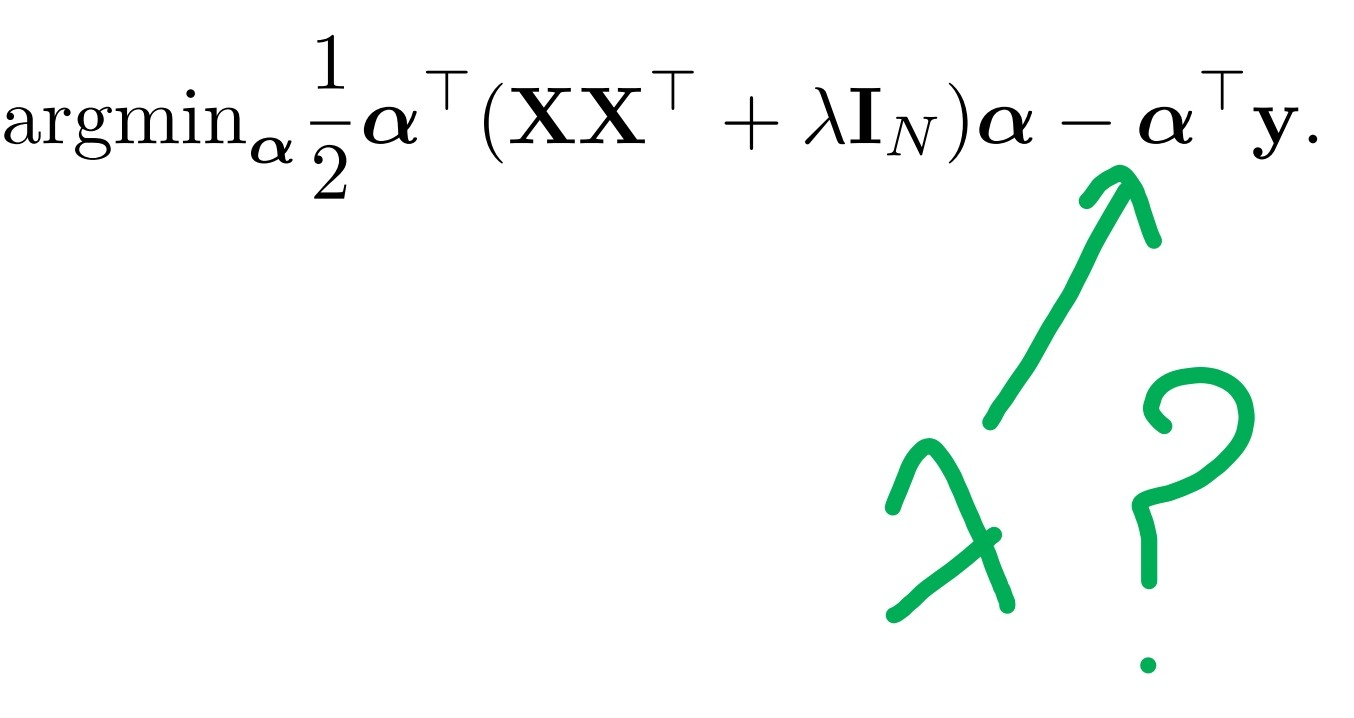### Possible error in pb11 exam2018

Hello,
Here is the formula of the kernelized ridge regression, shouldn't there be the lambda term acting as penalizer?Top comment

I think that the formula is correct. If you expand the left term you find 1/2 lambda a.T @ a which is the regularization term

I am sorry I didn't understand your answer, is there another explanation because I checked and the formula always mentions an extra lambda.

The regularization term $$\lambda \Vert \alpha\Vert^2$$ is hidden in (and is exactly) the term $$\alpha^\top \lambda I_N \alpha$$. However the matrix $$X$$ needs to be full rank for this problem to be equivalent to ridge regression.

Could I ask how to get this formula? I am sorry that I cannot deduce this.

Page 1 of 1